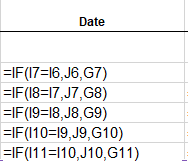cancel
Showing results for
Did you mean:

View discussion in a popup

Hi @gaugemf ,

If you copy the formula J8

IF(I8=I7,J7,G8)

to J7

IF(I8=I7,J7,G8)

and then preface J7 with an '=' -- you'll certainly get a circular reference (as it did when I checked).

You want to enter the formula in J8 exactly as:

=IF(I8=I7,J7,G8)

with the leading '=', then copy the contents of J8  down the length of the column.  you should end with something like this (but the length of the column)Essentially, the logic is to compare the current row of COL I with the previous row of column I (that is "Is this (CURRENT) row's Payment the same as the previous (I-1)?") if so Use the SAME DATE (J-1), otherwise (I'm on a new payment) get the new date (G(same row)))

Apologies for the confusion, but hope that helps.

Sitemap